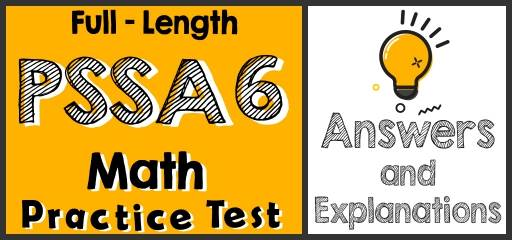Did you take the 6th Grade PSSA Math Practice Test? If so, then it’s time to review your results to see where you went wrong and what areas you need to improve.

Plug in the value of $$x$$ and $$y$$ and use the order of operations rule. $$x=2$$ and $$y=-3$$
$$5(4x-3y)-7y^2=5(4(2)-3(-3))-7(-3)^2=5(8+9)-7(9)=5(17)-63=85-63=22$$
For one hour he earns $18, then for t hours he earns$18t. If he wants to earn at least $78, therefore, the number of working hours multiplied by 18 must be equal to 78 or more than 78. $$18t≥78$$ 3- Choice B is correct $$(108-(3×9))÷9=9^3÷81=9$$ 4- Choice B is correct The ratio of boys to girls is 3 ∶ 5. Therefore, there are 3 boys out of 8 students. To find the answer, first, divide the total number of students by 8, then multiply the result by 3. $$240÷8=30 ⇒ 30×3=90$$ 5- Choice A is correct Probability$$=\frac{number \space of \space desired \space outcomes}{number \space of \space total \space outcomes}=\frac{9}{9+15+14+16}=\frac{9}{54}\frac{1}{6}=0.16$$ The Absolute Best Book to Ace the PSSA Math Test 6- Choice D is correct Let’s compare each fraction: $$\frac{2}{3}<\frac{3}{4}<\frac{7}{9}<\frac{4}{5}$$ Only choice D provides the right order. 7- Choice B is correct Let $$y$$ be the width of the rectangle. Then; $$14×y=84→y=\frac{84}{16}=6$$ 8- Choice B is correct $$4×\frac{5}{16}=\frac{20}{16}=1.25$$ A. $$1.25>2$$ B. $$1<1.25<2$$ This is the answer! C. $$\frac{3}{8}=1.25$$ D. $$1.25=2^2$$ 9- Choice B is correct In any rectangle, The measure of the sum of all the angles equals $$180^\circ$$. 10- Choice C is correct $$\frac{824}{17}=48.5$$ ## Best 6th Grade PSSA Math Prep Resource for 2022 11- The answer is $$7^2$$. $$588=2^2×3^1×7^2$$ 12- Choice B is correct The area of the trapezoid is: Area $$=\frac{base \space 1+base \space 2}{2}×height=\frac{12+10}{2}x=A→ 11x=A→x=\frac{A}{11}$$ 13- Choice B is correct $$\frac{72}{8}=9, \frac{648}{72}=9, \frac{5,832}{648}=9$$, Therefore, the factor is 9. 14- Choice C is correct Simplify each option provided. A. $$13-(3×6)+(7×(-6))=13-18+(-42)=-5-42=-47$$ B. $$(\frac{25}{400})+(\frac{7}{50})=\frac{25}{400}+\frac{56}{400}=\frac{81}{400}$$ C. $$((22×\frac{30}{6})-(7×\frac{144}{12}))×\frac{18}{2}=(110-84)×9=26×9=234$$ (this is the answer) D. $$(\frac{6}{24}+\frac{12}{33})-50=(\frac{1}{4}+\frac{1}{3})-50=(\frac{3}{12}+\frac{4}{12})-50=\frac{7}{12}-50=\frac{-593}{15}$$ 15- Choice D is correct To find the discount, multiply the number ($$100\%$$- rate of discount) Therefore; $$450(100\%-16\%)=450(1-0.16)=450-(450×0.16)$$ 16- Choice A is correct 1,400 out of 11,900 equals to $$\frac{1,400}{11,900}=\frac{200}{1,700}=\frac{2}{17}$$ 17- Choice C is correct The opposite of Nicolas’s integer is $$25$$. So, the integer is $$-25$$. The absolute value of $$25$$ is also $$25$$. 18- Choice B is correct Volume of a box = length × width × height = 7 × 4 × 12 = 336 19- Choice C is correct 1 yard = 3 feet, Therefore, $$33,759 ft×\frac{1 \space yd}{3 \space ft}=11,253$$ yd 20- Choice B is correct $$16\%$$ of the volume of the solution is alcohol. Let $$x$$ be the volume of the solution. Then: $$16\%$$ of $$x=38$$ ml ⇒ $$0.16x=38 ⇒ x=38÷0.16=237.5$$ The Most Comprehensive Review for 6th-Grade Students ## Related to This Article ### More math articles ### What people say about "Full-Length 6th Grade PSSA Math Practice Test-Answers and Explanations - Effortless Math: We Help Students Learn to LOVE Mathematics"? No one replied yet. X 51% OFF Limited time only! Save Over 51% SAVE$15
It was $29.99 now it is$14.99